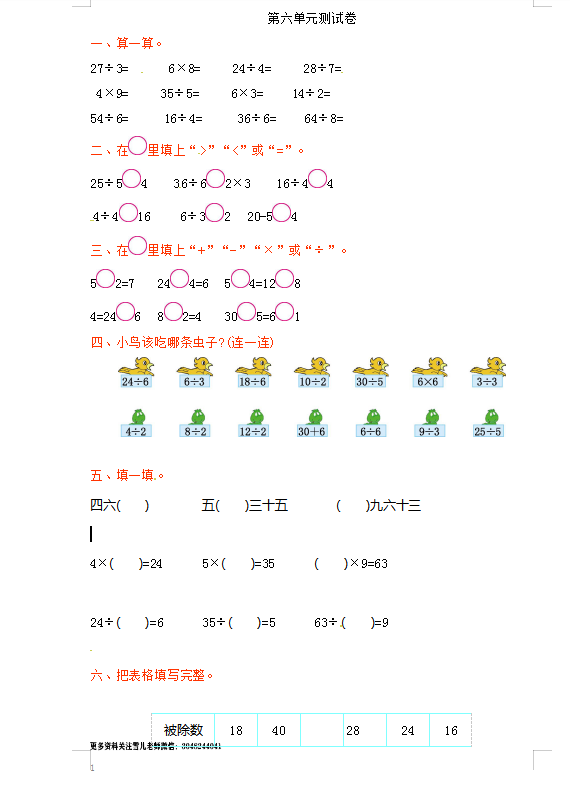27÷3=　　  6×8=　 　24÷4=　 　28÷7=

4×9=　　   35÷5=         6×3=　      14÷2=

54÷6=　      16÷4=          36÷6=      64÷8=